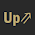# UPSC APFC Solved Question Paper- 10/01/2016

 Question Answer The devices that work with computer systems as soon as they are connected are described as Plug-N-Play Which one of the following software applications would be the most appropriate for performing numerical and statistical calculations? Spreadsheet Consider the following statements on Global Positioning System (GPS): GPS allows accurate time- stamping on ATM transactions GPS relies on a set of satellites for transferring signals worldwide. Consider the following statements: Cellular technology evolves in stages called Generation (G), where 2.    2G technology has two standards CDMA and GSM There are two circle of radii r1 and r2 (r1 < r2). The area of bigger circle is 693/2 cm2. The difference of their circumference is 22cm. What is the sum of the diameters of two circles? 35 cm. Numbers a1, a2, a3, a4, a5 ……… a24 are in arithmetic progression and a1 + a5 + a10 + a15 + a20 + a24 =225 The value of a1 + a2 + a3 + a4 + a5 + ………+ a23 + a24 is 900
Please report if you find any errors in the questions.
Keep watching the spaceThanks for reading UPSC APFC Solved Question Paper- 10/01/2016

←Previous
« Prev Post
Next→
Next Post »

1.Thanks sir..... But stridhan ke answer pe kuch doubt ho raha hai mujhe.... I think 1&3 are correct.... Plz suggest.

1.Yes Abhi, you are right.
Thanks to bring this to our attention.

2.I think same for stridhan 1&3 are correct

1.3.